Phone: +421 332 60 10 29
Mon-Fri: 9 a.m.- 6 p.m.
Cart
0 Items / €0.00

# Encoder’s PPR, CPR and LPR as resolution value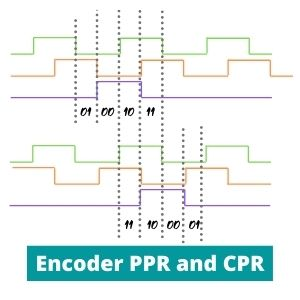CONTENTS:

## Encoder PPR and resolution

Encoder PPR (pulses per revolution) means how many pulses can pass through the encoder disk to the receiver in one full rotation. Maximum possible amount of encoder pulses determines its resolution.

A rotary encoder measures the displacement angle of its own shaft, which is connected to the measurement object (e.g. motor shaft).

There are two main sensing types, depending on position coding:. Each of them has specific tasks.

The incremental encoders measure the offset from a starting point using special signals (encoder pulses). They also can be used to determine the shaft rotation speed, acceleration and direction.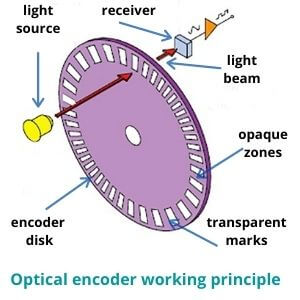Absolute sensors indicate a specific angle of displacement at the current moment (in degrees). Its disk is divided into sectors in which the position is already encoded. So, there is no need to count anything.

Let’s consider how such impulses occur.

## Pulse encoder

There are different technologies and types of encoders, but the principle of signal generation is mostly the same. Therefore, for pulse definition we will talk about the most common incremental optical rotary encoder.

In the case of an optical rotary sensor, it uses a disk, a light source and a receiver. The disk is attached to the shaft, between the receiver and the source. There are marks located on the disk. The light beam passes through marks (windows) to the receiver and does not pass through opaque areas.

All such marks on the disk are identical. Hence, signal from each of them will be the same too. Therefore, the device counts only amount and frequency of such signals. You can read how the resolution is calculated for other types (linear, rotary absolute, etc.) here: Encoder resolution.

The receiver captures all incoming light beams. Then device generates a digital or analog output signal, sending it to control systems or a PLC.

One of the most common used angular sensors are quadrature encoders. They send the signal with results as square waves.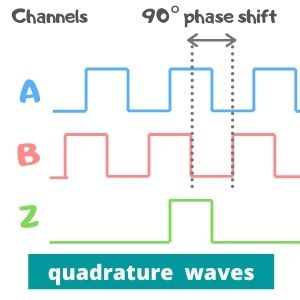Multiple waves can come in parallel from multiple output channels.  Such approach is used to determine the direction and for greater accuracy. The most common incremental encoders use three channels:

1. Channel A
2. Channel B
3. Channel Z

Channel A determines the number of pulses. That is, it provides information about the number of hits of the light beam into the receiver.

Channel B is identical to the Channel A. Channel B is identical to channel A, but is used to determine the direction of rotation (clockwise or counterclockwise). However, the square waves here are shifted by one phase of 90 degrees to channel A waves.  By comparing the differences between the channel A and B signals, the direction is determined.

Channel Z is needed for notification of the passage of a certain point or zero point. It is also called encoder Z pulse (zero or index pulse). It is necessary when you need a point that defines a certain displacement.

## Encoder PPR calculation

The signals from all three channels can be visually represented using the graph. The picture shows a normal quadrature pulses.

The signal forms high state (1) when the light beam reaches the receiver and low state (0) when it hits the opaque areas. That is, there are only two values, so the waves have such form.

Such output signals are the same for all quadrature encoders. However, each manufacturer can use a different method of analyzing the output signals.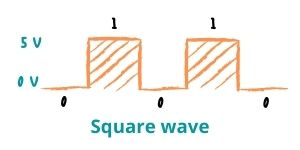The most common resolution values used in rotary quadrature sensors are:

• PPR
• CPR
• LPR

Now let's look what is encoder ppr, cpr and lpr apart.

## Rotary encoder pulses per revolution

PPR is the most common encoder resolution value. Pulse here means a period of time during which the signal is at its highest point (1). For example, if the light beam passed through 5 marks and reached the receiver 5 times, then we get 5 such pulses. If there are 360 marks located on the disk, then encoder can generate a maximum of 360 pulses per revolution (PPR).

So, the PPR in encoder resolution tells how much maximum pulses can produce an encoder during a single full rotation of its disk.

Be aware! Some manufacturers use the term PPR as the number of periods per turn. A period in this case is one full form of encoder signal cycle, including high and low states (1 and 0). In this case, the encoder may have a different resolution value, but it is also called as PPR.

## Encoder CPR meaning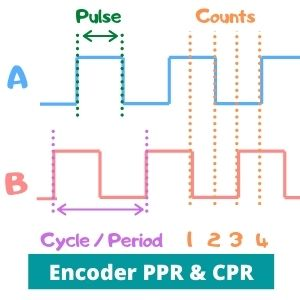CPR (counts per revolution) defines the ratio of states between two channels (Ch. A and Ch. B) at 4 different points. How does a cpr quadrature encoder work? Using this method, the encoder calculates every signal edge in two square waves’ graphs (raising A (1), falling A (0), raising B (1), falling B (0).

Digital output signal value in that case has form of a pair of digits (01, 11, 00, and 10). This is the smallest value that the quadrature encoder can measure.

Be aware! Under CPR acronym, manufacturers can mean the resolution in cycles per revolution. In this case, it is the same as periods per revolution. The word «cycle» denotes to the electrical cycle of the signal.

Don't be confused this is in four times higher than counts per revolution. You can convert counts into pulses or cycles by multiplying result in four times (because it has 4 points).

LPR (lines per revolution) is the most rarely used value. Lines here refers to the number of marks on the disk. Therefore, it is the same as number of encoder pulses and PPR value.

Conclusion: Pulses and square waves for encoders occurs by the same method. However, there are many approaches for calculating the results. Some manufacturers may count resolution in one way; some may count it by another. You should always check the datasheet and specification carefully.

We are official distributors of Eltra encoders. In our website, you will find a large catalog of eltra quadrature encoders. If you have questions or need eny help with the choice feel free to call us or send a request!

Name*
Email
9 + ? = 17